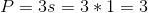# SSAT Elementary Level Math : How to find the perimeter of a triangle

## Example Questions

### Example Question #21 : How To Find The Perimeter Of A Triangle

What is the PERIMETER of the triangle in the figure?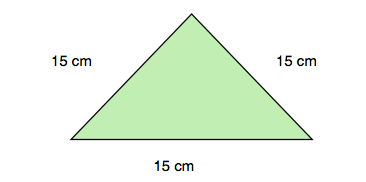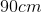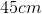30 cm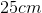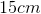Explanation:

Because the question asks you to find the PERIMETER of the triangle, you are looking to figure out the distance around the outside of the triangle. You find the perimeter of triangles simply by adding the length of the three sides together. So the correct answer here is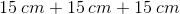, or.

### Example Question #22 : How To Find The Perimeter Of A Triangle

What is the PERIMETER of the triangle in the figure?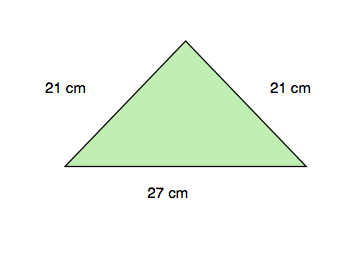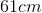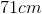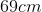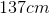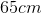Explanation:

Because the question asks you to find the PERIMETER of the triangle, you are looking to figure out the distance around the outside of the triangle. You find the perimeter of triangles simply by adding the length of the three sides together. So the correct answer here is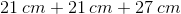, or.

### Example Question #23 : How To Find The Perimeter Of A Triangle

What is the PERIMETER of the triangle in the figure?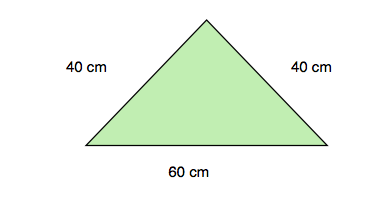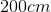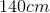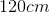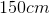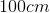Explanation:

Because the question asks you to find the PERIMETER of the triangle, you are looking to figure out the distance around the outside of the triangle. You find the perimeter of triangles simply by adding the length of the three sides together. So the correct answer here is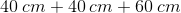, or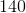cm.

### Example Question #51 : Triangles

Find the perimeter of an equilateral triange whose side length is 8.Explanation:

To find perimeter of an equilateral triangle, simply multiply the side length by 3. Thus,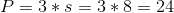### Example Question #25 : How To Find The Perimeter Of A Triangle

Find the perimeter of an equilateral triangle whose side length is 6.Explanation:

To find perimeter of an equliateral triangle, simply multiply the side length by 3. Thus,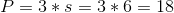### Example Question #1 : Recognize And Draw Shapes: Ccss.Math.Content.2.G.A.1

Which shape has onlysides?

Triangle

Cube

Pentagon

Hexagon

Square

Triangle

Explanation:

A triangle hassides.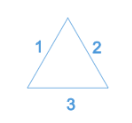### Example Question #27 : How To Find The Perimeter Of A Triangle

Find the perimeter of an equilateral triangle whose side length is 9.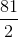Explanation:

To solve, simply use the following formula for the perimeter of an equilateral triangle. Thus,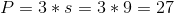### Example Question #12 : Recognize And Draw Shapes: Ccss.Math.Content.2.G.A.1

What shape has onlyangles?

Hexagon

Cube

Pentagon

Square

Triangle

Triangle

Explanation:

A triangle is the only shape withangles.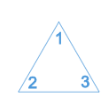### Example Question #28 : How To Find The Perimeter Of A Triangle

If you have an equilateral triangle and the length of one side is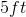, what is the perimeter?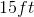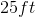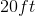Not enough information is given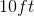Explanation:

An equilateral triangle has three equal sides.  To find the perimeter of a triangle, you add up all the sides.  Your final answer would then be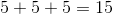.

### Example Question #29 : How To Find The Perimeter Of A Triangle

Find the perimeter of an equilateral triangle with side length 1.